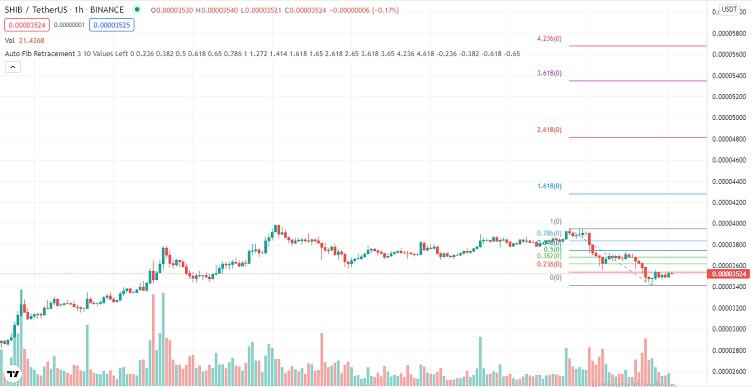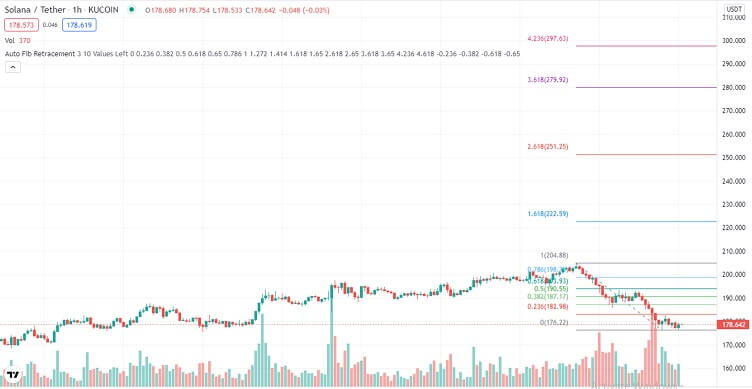# Dogecoin, Shiba Inu & Solana Technical Analysis – 29th Dec

## Cryptocurrencies bounce back after crashing on Tuesday

Last Updated December 29th 2021
• Dogecoin makes a strong reversal off the 61.8% Fibonacci support at \$0.1740. It needs to push through the 50.0% Fib resistance at \$0.1781 for an uptrend to be confirmed.
• Shiba Inu bulls struggle at \$0.00003545 on the 38.2% Fibonacci resistance.
• Solana bears are still in control as they target 78.6% Fibonacci support at \$175.79

## Dogecoin

Dogecoin was bearish for the better part of Tuesday. By the end of the day, it was down by 12%.

Dogecoin started Tuesday trading bearishly, and within the first hour of the day, had crossed through the 23.6% Fibonacci support at \$0.1874.

The selloff was quite strong, and just before daybreak, DOGE had broken the 38.2% Fibonacci support at \$0.1823 and tested the 50.0% Fibonacci support at \$0.1781.

The 50.0% Fibonacci support proved to be quite strong, and Dogecoin bounced off it. By noon, Dogecoin had retested the 38.2% Fibonacci, then resistance at \$0.1823.

The 38.2% Fibonacci resistance proved to be quite strong, and with the broader market bearish, the 50.0% Fibonacci support at \$0.1781 gave way.

By early evening, Dogecoin had hit the 61.8% Fibonacci support at \$0.1740. Dogecoin bounced off this support, and by the end of the day, had retested the 50.0% Fibonacci, now resistance at \$0.1781.

Dogecoin started Wednesday trading bearish after bulls failed to push through the 50.0% Fibonacci resistance at \$0.1781.

When going to press, Dogecoin was trading at \$0.1740 at the 61.8% Fibonacci support.### A glance at the day ahead

The key levels to watch are the 50.0% Fibonacci resistance at \$0.1781 and the 61.8% Fibonacci support at \$0.1740.

If bulls take control and push Dogecoin through the 50.0% Fibonacci resistance at \$0.1781, the 38.2% Fibonacci resistance at \$0.1823 would come into focus. If the 38.2% Fib resistance breaks, prices above \$0.1857 could be seen in the day.

However, if bears take control and push Dogecoin through the 61.8% Fibonacci support, the next key level to watch would be the 78.6% Fibonacci support at \$0.1682.

If Dogecoin pushes through the 78.6% Fibonacci support, prices below \$0.164 could be attainable within the day.

On the other hand, if volumes drop, Dogecoin could trade between the 50.0% Fibonacci resistance at \$0.1781, and the 61.8% Fibonacci support at \$0.1740.

### A glance at the technicals

Key resistance: 50.0% Fibonacci at \$0.1781

Key support: 61.8% Fibonacci at \$0.1740

## Shiba Inu

Shiba Inu was bearish for the better part of Tuesday. By the end of the day, SHIB was down by 12.5%.

Shiba Inu started Tuesday trading bearish, a continuation of the selloff that started in late Monday trading.

By the second hour of the day, Shiba Inu had pushed through the 23.6% Fibonacci support at \$0.00003716.

Just before daybreak, Shiba Inu came close to testing the 38.2% Fibonacci support at \$0.00003545.

However, the 38.2% Fibonacci support proved to be too strong for bears, and Shiba Inu bounced off it.

By mid-morning, Shiba Inu had tested the 23.6% Fibonacci, now resistance, at \$0.00003716.

However, with the broader market bearish, Shiba Inu bulls could not break the 23.6% Fibonacci support. What followed was a crash that saw Shiba Inu breach the 38.2% Fibonacci support, and test the 50.0% Fibonacci support at 0.00003410.

SHIB bounced off the 50.0% Fibonacci support, and by the end of the day, had retested the 38.2% Fibonacci, now resistance at \$0.00003545.

Shiba Inu started Wednesday trading at the 38.2% Fibonacci resistance. However, volumes were low, and at the time of going to press, SHIB was yet to push through this resistance.### A glance at the day ahead

The key levels to watch are the 38.2% Fibonacci resistance at \$0.00003545 and the 50.0% Fibonacci support at \$0.00003408.

If bulls take control and push Shiba Inu through the 38.2% Fibonacci resistance at \$0.00003545, the 23.6% Fibonacci resistance at \$0.00003716 would come into focus. If the 23.6% Fibonacci resistance at \$0.00003716 is broken, prices above \$0.00003901 could be seen in the day.

However, if the 50.0% Fibonacci support at \$0.00003408 is broken, prices below \$0.00003321 could be seen within the day.

On the other hand, if volumes drop, Shiba Inu could trade between the 38.2% Fibonacci resistance at \$0.00003545, and the 50.0% Fibonacci support at \$0.00003408.

### A glance at the technicals

Key resistance:  38.2% Fibonacci at \$0.00003545

Key support: 50.0% Fibonacci at \$0.00003408

## Solana

Solana was bearish for the better part of Tuesday, and by the end of the day, was down by 10.27%.

Solana started Tuesday trading bearish, a continuation of the selloff that started in late Monday trading.

Within three hours of trading, Solana had breached the 38.2% Fibonacci support at \$190.73 and tested the 50.0% Fibonacci support at \$186.31.

After such heavy selling, Solana bulls attempted to take control at \$186.31 on the 50.0% Fibonacci support.

By mid-morning, Solana bulls had hit and briefly pushed through the 38.2% Fibonacci resistance at \$190.73.

However, with the broader market overall bearish, bulls could not sustain momentum through the 38.2% Fibonacci resistance at \$190.73.

The selloff accelerated, and by early evening, Solana had tested the 78.6% Fibonacci support at \$175.79.

It bounced off this support, and within an hour, had tested the 61.8% Fibonacci resistance at \$181.96.

Bulls lost momentum again, weighed down by selling pressure in the broader market. By the end of the day, Solana was close to retesting the 78.6% Fibonacci support at \$175.79.

Solana started Wednesday trading bullish after bears lost momentum slightly above the 78.6% Fibonacci support. At the time of going to press, bulls were still in control, but volumes were low.### A glance at the day ahead

The key levels to watch are the 61.8% Fibonacci resistance at \$181.96 and the 78.6% Fibonacci support at \$175.79.

If bulls take control and push Solana through the 61.8% Fibonacci resistance, the next key level to watch would be the 50.0% Fibonacci resistance at \$186.31. If the 50.0% Fibonacci resistance is broken, prices above \$190 could be seen within the day.

However, if the 78.6% Fibonacci support at \$175.79 is broken, the next key level to watch would be the multi-week support at \$167.86. If this support is broken, prices below \$165 could be seen in the day.

On the other hand, if volumes drop, Solana could trade between the 61.8% Fibonacci resistance at \$181.96 and the 78.6% Fibonacci support at \$175.79.

### A glance at the technicals

Key resistance: 61.8% Fibonacci at \$186.96

Key support: 78.6% Fibonacci at \$175.79